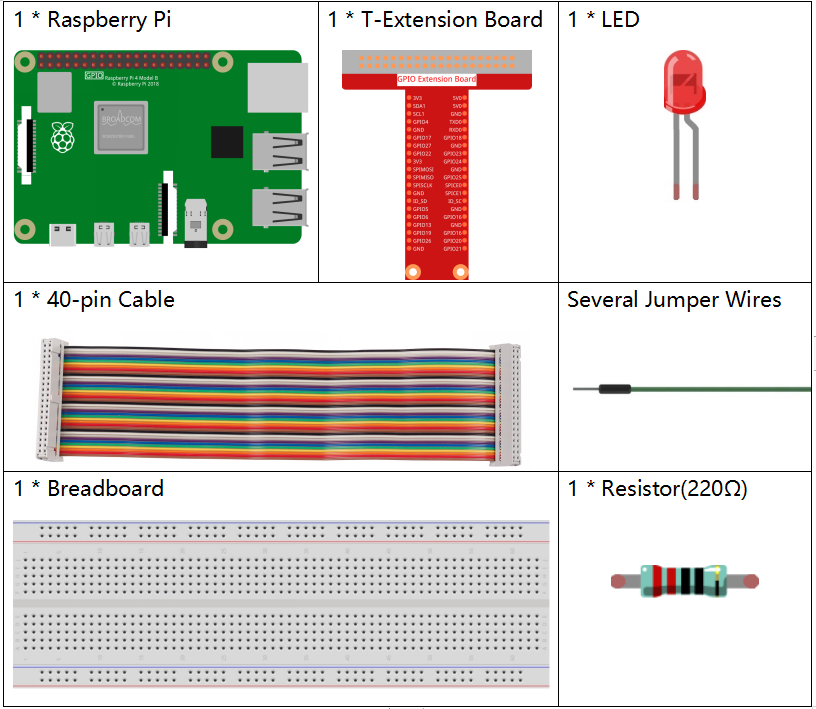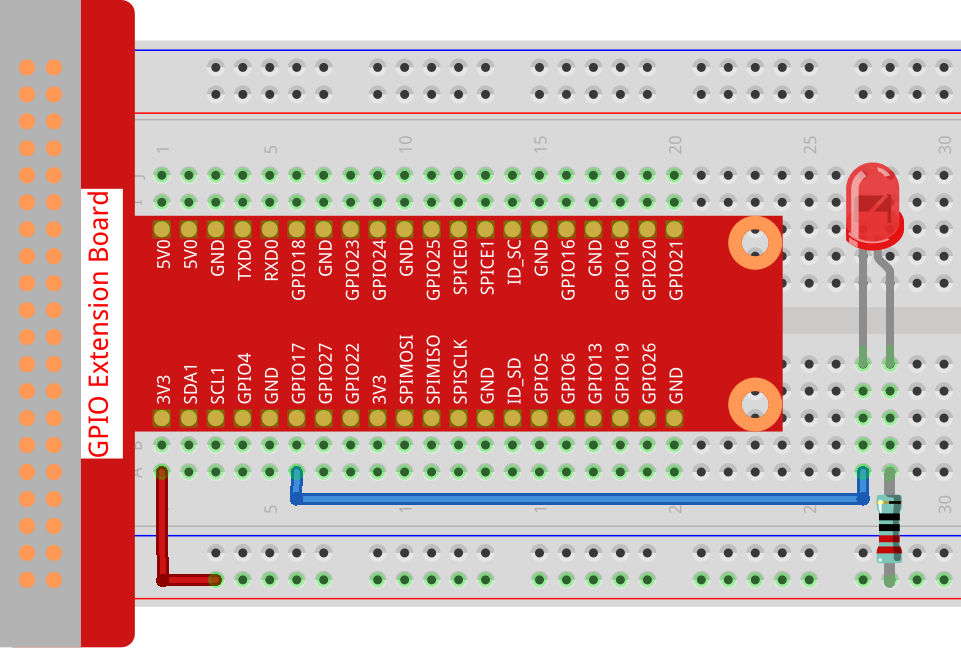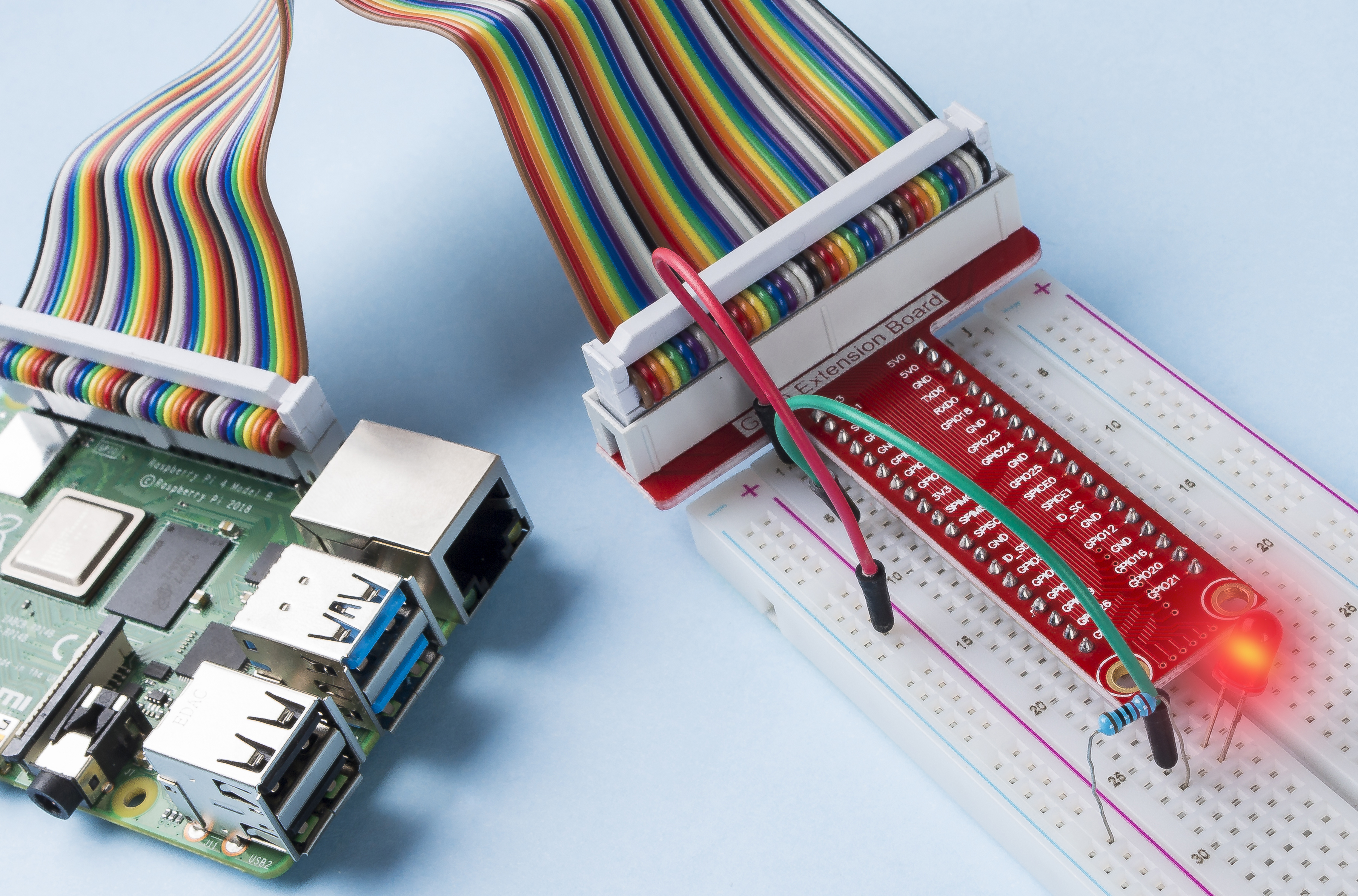## Introduction¶

In this project, we will learn how to make a blinking LED by programming. Through your settings, your LED can produce a series of interesting phenomena. Now, go for it.

## Components¶## Schematic Diagram¶

In this experiment, connect a 220Ω resistor to the anode (the long pin of the LED), then the resistor to 3.3 V, and connect the cathode (the short pin) of the LED to GPIO17 of Raspberry Pi. Therefore, to turn on an LED, we need to make GPIO17 low (0V) level. We can get this phenomenon by programming.## Experimental Procedures¶

Step 1: Build the circuit.Step 2: Go to the folder of the code.

```cd ~/davinci-kit-for-raspberry-pi/nodejs/
```

Note

Change directory to the path of the code in this experiment via `cd`.

Step 3: Run the code

```sudo node blink.js
```

Note

Here sudo - superuser do, and python means to run the file by Python.

After the code runs, you will see the LED flashing.

Step 4: If you want to edit the code file blink.js, press `Ctrl + C` to stop running the code. Then type the following command to open blink.js:

```nano blink.js
```

Note

nano is a text editor tool. The command is used to open the code file blink.js by this tool.

Press `Ctrl+X` to exit. If you have modified the code, there will be a prompt asking whether to save the changes or not. Type in `Y` (save) or `N` (don’t save).

Then press `Enter` to exit. Type in nano blink.js again to see the effect after the change.

Code

The following is the program code:

```const Gpio = require('pigpio').Gpio;
const led = new Gpio(17,{mode: Gpio.OUTPUT});

var led_state = 0;

led.digitalWrite(led_state);
led_state = !led_state;
}

```

Alternatively, write the code as a more js-specific arrow function:

```const Gpio = require('pigpio').Gpio;
const led = new Gpio(17,{mode: Gpio.OUTPUT});

var led_state = 0;

setInterval(() => {
led.digitalWrite(led_state);
led_state = !led_state;
}, 300);
```

Code Explanation

```const Gpio = require('pigpio').Gpio;
```

Import the Gpio constructor in the pigpio package in this way, and then define a constant `Gpio` to represent this constructor.

With a variety of constructor, we can use js to control electronic devices. `pigpio` can be used to implement fast GPIO, PWM, servo control, state change notification and interrupt handling.

```const led = new Gpio(17,{mode: Gpio.OUTPUT});
```

The `new` keyword is used to construct instantiated objects of the class.

Connect the LED to the GPIO17 of the T-shaped expansion board, set the LedPin mode to output and assign it to the constant `led`, that is, construct a GPIO17 object `led`, and its mode is the output mode.

There are two ways to number the IO pins on the Raspberry Pi: BOARD number and BCM number. In our project, we use the BCM number. You need to set each channel used as input or output.

```function blink_led(){
led.digitalWrite(led_state);
led_state = !led_state;
}

```

The `setInterval()` method can call functions or compute expressions with a specified period (in milliseconds). Here we change the operating state of the LED with a period of 300ms.

The `gpio.digitalWrite(level)` method sets the gpio level to 1 or 0.

```var led_state = 0;

setInterval(() => {
led.digitalWrite(led_state);
led_state = !led_state;
}, 300);
```

Rewrite the code as an arrow function to make the code shorter.

## Phenomenon Picture¶